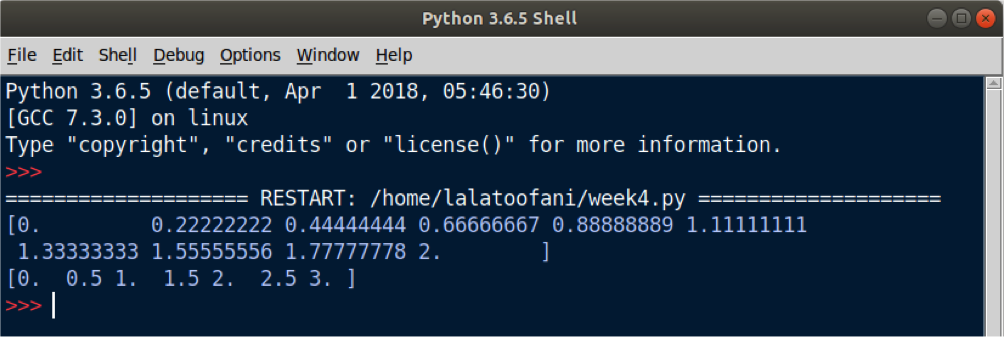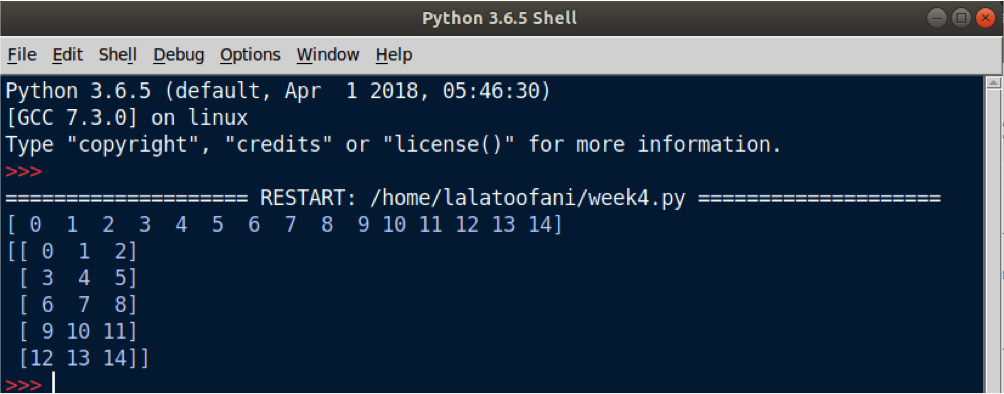## NumPy Module In Python

##### In this part of Learning Python we Cover Numpy Module in python
Written by |10-May-2019 | 0 Comments | 283 Views

Previously we have seen the introduction of NumPy and some of the basics of NumPy along with the installation. Now we will see some more basics to understand more functionality of the NumPy module.

Identity Matrix:

The identity matrix is a type of matrix in which all the elements of the principal diagonal matrix are one, and all the value other than a diagonal value are zero.

To initialize the identity matrix eye() function is used in NumPy. The eye function takes only one parameter which is used as both rows and columns because for identity matrix it is compulsory that the matrix should be square.

Following is the method to initialize the identity matrix:

` import numpy as np`

`print (" Initializing by eye function ")`
`print (np.eye(7))`

If we run the above code following output will be generated:

 Output   Initializing by eye function [[1. 0. 0. 0. 0. 0. 0.]  [0. 1. 0. 0. 0. 0. 0.]  [0. 0. 1. 0. 0. 0. 0.]  [0. 0. 0. 1. 0. 0. 0.]  [0. 0. 0. 0. 1. 0. 0.]  [0. 0. 0. 0. 0. 1. 0.]  [0. 0. 0. 0. 0. 0. 1.]]

In the above function, we use eye function and give it value seven as a parameter, and in return, an array is initialized having seven rows and seven columns. All the value in diagonal are one, and all the values other than diagonal are given the value of zero.

# Some Basics of NumPy:

• ndarray.ndim: It returns the number of axis or dimension of an array. It tells whether an array is one dimensional, two dimensional or n-dimensional array.
• ndarray.shape: This function will return the size means the number of columns and the number of rows will be returned. The rows and column will be returned as a tuple value.
•  ndarray.size: By using this function, the total number of elements in an array will be returned.
• ndarray.dtype: This function will describe the type of element in the array.

The coding implementation of the above functionality is as follows. Suppose we have initialized the one's array of 3 dimension now we will check all the above functionality one by one in our coding editor:

`import numpy as np`

`array = np.ones((3,4,5))`
`print(array)`

`print("Array dimension")`
`print(array.ndim)`

`print("Rows and columns in array")`
`print(array.shape)`

`print("Number of elements in an array")`
`print(array.size)`

`print("Data type of an array")`
`print(array.dtype)`

The output of the above example is as follows:

 [[[1. 1. 1. 1. 1.]   [1. 1. 1. 1. 1.]   [1. 1. 1. 1. 1.]   [1. 1. 1. 1. 1.]]    [[1. 1. 1. 1. 1.]   [1. 1. 1. 1. 1.]   [1. 1. 1. 1. 1.]   [1. 1. 1. 1. 1.]]    [[1. 1. 1. 1. 1.]   [1. 1. 1. 1. 1.]   [1. 1. 1. 1. 1.]   [1. 1. 1. 1. 1.]]] Array dimension 3 Rows and columns in array (3, 4, 5) Number of elements in an array 60 Data type of an array float64

Here we can see that dimensions are 3, rows columns concerning dimensions are (3,4,5). If we multiple 3x4x5 we get the 60, so the total number of elements in an array is 60 and data type of each element is a float.

Linespace:

Suppose we have a task to generate n numbers between two given numbers. In actual it is a long task, but NumPy makes it very easy. Suppose we want to get ten numbers between zero and one so NumPy linespace method will create an array of ten elements starting from zero and ending to one and in between there will be equal intervals.

Following is the code for linespace:

`import numpy as np`

`a = np.linspace(0,2,10)`
`print(a)`

`b = np.linspace(0,3,7)`
`print(b)`

The above written is the code for the linspace method. It takes three parameters. The parameter is where to start, the second one is the ending point, and the third one is the number of intervals. The variable took ten intervals, and variable b took seven intervals.

Following is the output generated by this code.

 output a [0.         0.22222222 0.44444444 0.66666667 0.88888889 1.11111111  1.33333333 1.55555556 1.77777778 2.        ]   output b [0.  0.5 1.  1.5 2.  2.5 3. ]Figure 1

The first output generates ten intervals and second output generates seven intervals.

Reshape:

Reshaping method is used to convert or to manipulate the rows and columns of the NumPy array. This function is mostly used when doing image processing to change the number of pixels or when subjected to neural network than reshaping is used to make the image acceptable for the network.

Following is the code for reshaping:

`import numpy as np`

`a = np.arange(15)`
`print(a)`

`print(a.reshape(5,3))`

After importing the module, we make a variable and, in that variable, we initialize the NumPy array of 1 row and 15 columns. Then we reshape it by five rows and three columns,

The output is as follows:

 Output of arange function [ 0  1  2  3  4  5  6  7  8  9 10 11 12 13 14]   Output after reshaping the numpy array [[ 0  1  2]  [ 3  4  5]  [ 6  7  8]  [ 9 10 11]  [12 13 14]]Figure 2

Mathematical Operation:

We can do all sort of mathematical operations in NumPy like addition, subtraction, multiplication, square, exponential, dot matrix, square root, etc.

Following are the example code of doing mathematical operation using NumPy which otherwise could be a lengthy process when data is massive.

`import numpy as np`

`a = np.array([1,2,3,4])`
`b = np.array([5,6,7,8])`

`print (b-a)`
`print (b*a)`
`print (b+a)`
`print (a*2)`
`print (b*2)`
`print (b**2)`
`print (np.exp(b))`
`print (np.sqrt(a))`
`print (np.sin(a))`
`print (np.cos(b))`
`print (a.dot(b))`

The output of the above code is as follows:

 Subtraction [4 4 4 4]   Multiplication [ 5 12 21 32]   Addition [ 6  8 10 12]   Multiplication [2 4 6 8] [10 12 14 16]   Square [25 36 49 64]   Exponential [ 148.4131591   403.42879349 1096.63315843 2980.95798704]   Square root [1.         1.41421356 1.73205081 2.        ]   Sin value [ 0.84147098  0.90929743  0.14112001 -0.7568025 ]   Code value [ 0.28366219  0.96017029  0.75390225 -0.14550003]   Dot product of a matrix 70